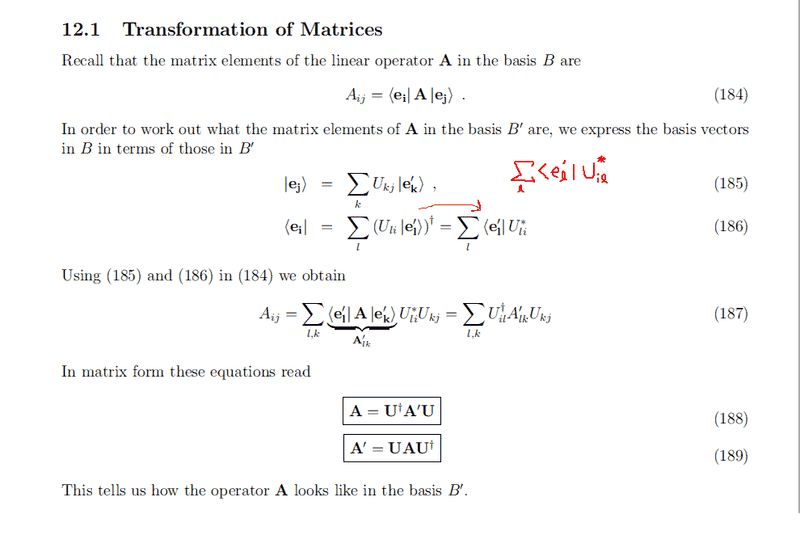# Matrix Transformation of operator from basis B' to B

• unscientific

## Homework Statement

Hi guys, actually this isn't a homework question, but rather part of the working in a textbook on Linear Algebra.## The Attempt at a Solution

I'm not sure why it's U*li instead of U*il. Shouldn't you flip the order when you do a matrix transpose?

you need to be careful with this stuff. There are two different things going on really.
1) taking the conjugate transpose of the equation
##U_{li} | \boldsymbol{e}_l' >##
Now here, ##|\boldsymbol{e}_l' >## is a vector, so something interesting does happen to it, and it becomes ##<\boldsymbol{e}_l' |## OK. And then what about ##U_{li}## is it a vector, or an operator, or just a scalar? And therefore what happens to it when you are taking the conjugate transpose of the equation?

2)Something different is the equation: ##U_{li}^{*}=U_{il}^{\dagger}## Here, they are defining the components of the operator ##\boldsymbol{U}^{\dagger}## in relation to the components of the operator ##\boldsymbol{U}## i.e. they are not actually taking the conjugate transpose of ##U_{il}##

It is important to keep the distinction between the actual operators themselves, and the components of the operator.

Last edited:
•1 person
you need to be careful with this stuff. There are two different things going on really.
1) taking the conjugate transpose of the equation
##U_{li} | \boldsymbol{e}_l' >##
Now here, ##|\boldsymbol{e}_l' >## is a vector, so something interesting does happen to it, and it becomes ##<\boldsymbol{e}_l' |## OK. And then what about ##U_{li}## is it a vector, or an operator, or just a scalar? And therefore what happens to it when you are taking the conjugate transpose of the equation?

2)Something different is the equation: ##U_{li}^{*}=U_{il}^{\dagger}## Here, they are defining the components of the operator ##\boldsymbol{U}^{\dagger}## in relation to the components of the operator ##\boldsymbol{U}## i.e. they are not actually taking the conjugate transpose of ##U_{il}##

It is important to keep the distinction between the actual operators themselves, and the components of the operator.

I see. In a way, ##|\boldsymbol{e}_l' >## defines the direction whereas Uli is simply a complex number that has been pre-determined to tag along with that base vector. We cannot change Uli to U*il as that is reserved for a different complex number that is tagged along with a different base vector.

yeah, that's it. When we take the conjugate transpose of an equation involving complex numbers, then all that happens to those complex numbers is that they become their complex conjugate (since they are just a number). Often people can be sloppy with their terminology when they say that the complex conjugate of ##C_{ij}## is equal to ##C_{ji}^{*}## When they actually mean that if we have an operator ##\boldsymbol{C}##, then the complex conjugate of that operator is ##\boldsymbol{C}^{\dagger}## and the components of the two operators are given by relation above.